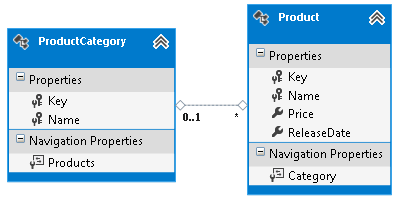# 自定义 Code First 约定

## 我们的模型

``````    using System;
using System.Collections.Generic;
using System.Data.Entity;
using System.Linq;

public class ProductContext : DbContext
{
static ProductContext()
{
Database.SetInitializer(new DropCreateDatabaseIfModelChanges<ProductContext>());
}

public DbSet<Product> Products { get; set; }
}

public class Product
{
public int Key { get; set; }
public string Name { get; set; }
public decimal? Price { get; set; }
public DateTime? ReleaseDate { get; set; }
public ProductCategory Category { get; set; }
}

public class ProductCategory
{
public int Key { get; set; }
public string Name { get; set; }
public List<Product> Products { get; set; }
}
``````

## 自定义约定简介

``````    public class ProductContext : DbContext
{
static ProductContext()
{
Database.SetInitializer(new DropCreateDatabaseIfModelChanges<ProductContext>());
}

public DbSet<Product> Products { get; set; }

protected override void OnModelCreating(DbModelBuilder modelBuilder)
{
modelBuilder.Properties()
.Where(p => p.Name == "Key")
.Configure(p => p.IsKey());
}
}
``````

``````    modelBuilder.Properties<int>()
.Where(p => p.Name == "Key")
.Configure(p => p.IsKey());
``````

IsKey 方法的一个有趣特征是具有累加性。 这意味着，如果在多种属性上调用 IsKey，它们都会成为组合键的一部分。 需要注意的是，为键指定多种属性时，还必须指定这些属性的顺序。 为此，可以调用 HasColumnOrder 方法，如下所示：

``````    modelBuilder.Properties<int>()
.Where(x => x.Name == "Key")
.Configure(x => x.IsKey().HasColumnOrder(1));

modelBuilder.Properties()
.Where(x => x.Name == "Name")
.Configure(x => x.IsKey().HasColumnOrder(2));
````````````    modelBuilder.Properties<DateTime>()
.Configure(c => c.HasColumnType("datetime2"));
``````

## 约定类

``````    public class DateTime2Convention : Convention
{
public DateTime2Convention()
{
this.Properties<DateTime>()
.Configure(c => c.HasColumnType("datetime2"));
}
}
``````

``````    protected override void OnModelCreating(DbModelBuilder modelBuilder)
{
modelBuilder.Properties<int>()
.Where(p => p.Name.EndsWith("Key"))
.Configure(p => p.IsKey());

}
``````

## 自定义特性

``````    [AttributeUsage(AttributeTargets.Property, AllowMultiple = false)]
public class NonUnicode : Attribute
{
}
``````

``````    modelBuilder.Properties()
.Where(x => x.GetCustomAttributes(false).OfType<NonUnicode>().Any())
.Configure(c => c.IsUnicode(false));
``````

``````    [AttributeUsage(AttributeTargets.Property, AllowMultiple = false)]
internal class IsUnicode : Attribute
{
public bool Unicode { get; set; }

public IsUnicode(bool isUnicode)
{
Unicode = isUnicode;
}
}
``````

``````    modelBuilder.Properties()
.Where(x => x.GetCustomAttributes(false).OfType<IsUnicode>().Any())
.Configure(c => c.IsUnicode(c.ClrPropertyInfo.GetCustomAttribute<IsUnicode>().Unicode));
``````

``````    modelBuilder.Properties()
.Having(x => x.GetCustomAttributes(false).OfType<IsUnicode>().FirstOrDefault())
.Configure((config, att) => config.IsUnicode(att.Unicode));
``````

## 配置类型

``````    private string GetTableName(Type type)
{
var result = Regex.Replace(type.Name, ".[A-Z]", m => m.Value + "_" + m.Value);

return result.ToLower();
}
``````

``````    modelBuilder.Types()
.Configure(c => c.ToTable(GetTableName(c.ClrType)));
``````

``````    private string GetTableName(Type type)
{
var pluralizationService = DbConfiguration.DependencyResolver.GetService<IPluralizationService>();

var result = pluralizationService.Pluralize(type.Name);

result = Regex.Replace(result, ".[A-Z]", m => m.Value + "_" + m.Value);

return result.ToLower();
}
``````

GetService 的通用版本是 System.Data.Entity.Infrastructure.DependencyResolution 命名空间中的扩展方法，需要在上下文中添加 using 语句才能使用它。

### ToTable 和继承

ToTable 的另一个重要方面是，如果将类型显式映射到给定表，则可以更改 EF 将使用的映射策略。 如果为继承层次结构中的每种类型调用 ToTable，并像上面那样将类型名称作为表的名称传递，则你将默认的每个层次结构一张表 (TPH) 映射策略更改为每个类型一张表 (TPT)。 对此进行说明的最佳方式是利用具体示例：

``````    public class Employee
{
public int Id { get; set; }
public string Name { get; set; }
}

public class Manager : Employee
{
public string SectionManaged { get; set; }
}
``````

``````    modelBuilder.Types()
.Configure(c=>c.ToTable(c.ClrType.Name));
``````1. 对层次结构中的每种类型使用同一表名调用 ToTable。
2. 仅在层次结构的基类（本例中为“员工”）上调用 ToTable。

## 执行顺序

``````    modelBuilder.Properties<string>()
.Configure(c => c.HasMaxLength(500));

modelBuilder.Properties<string>()
.Where(x => x.Name == "Name")
.Configure(c => c.HasMaxLength(250));
``````

Fluent API 和数据注释还可用于替代特定情况下的约定。 在以上示例中，如果已使用 Fluent API 设置属性的最大长度，则将其置于约定之前或之后，因为更具体的 Fluent API 将优先于更通用的配置约定。

## 内置约定

``````    modelBuilder.Conventions.AddBefore<IdKeyDiscoveryConvention>(new DateTime2Convention());
``````

``````    protected override void OnModelCreating(DbModelBuilder modelBuilder)
{
modelBuilder.Conventions.Remove<PluralizingTableNameConvention>();
}
``````